Want to share your content on R-bloggers? click here if you have a blog, or here if you don't.The apply functions in R are awesome (see this post for some lesser known apply functions). However, if you can use pure vectorization, then you’ll probably end up making your code run a lot faster than just depending upon functions like sapply and lapply. This is because apply functions like these still rely on looping through elements in a vector or list behind the scenes – one at a time. Vectorization, on the other hand, allows parallel operations under the hood – allowing much faster computation. This posts runs through a couple such examples involving string substitution and fuzzy matching.

### String substitution

For example, let’s create a vector that looks like this:

test1, test2, test3, test4, …, test1000000

with one million elements.

With sapply, the code to create this would look like:

```start <- proc.time()
samples <- sapply(1:1000000, function(num) paste0("test", num))
end <- proc.time()
print(end - start)

```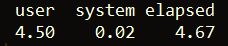As we can see, this takes over 4 1/2 seconds. However, if we generate the same vector using vectorization, we can get the job done in only 0.75 seconds!

```start <- proc.time()
samples <- paste0("test", 1:1000000)
end <- proc.time()
print(end - start)

```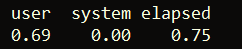Now, we can also use gsub to remove the substring test from every element in the vector, samples — also with vectorization:

```start <- proc.time()
nums <- gsub("test", "", samples)
end <-  proc.time()
print(end - start)

```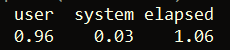This takes just over one second. In comparison, using sapply takes roughly eleven times longer!

```start <- proc.time()
nums <- sapply(samples, function(string) gsub("test", "", string))
end <-  proc.time()
print(end - start)

```### Fuzzy matching

Vectorization can also be used to vastly speed up fuzzy matching (as described in this post). For example, let’s use the stringi package to randomly generate one million strings. We’ll then use the stringdist package to compare the word “programming” to each random string.

```library(stringi)
library(stringdist)

set.seed(1)
random_strings <- stri_rand_strings(1000000, nchar("programming"),"[a-z]")

```

Now, let’s try using sapply to calculate a string similarity score (using default parameters) between “programming” and each of the one million strings.

```start <- proc.time()
results <- sapply(random_strings, function(string) stringsim("programming", string))
end <- proc.time()

print(end - start)

```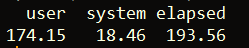As we can see, this takes quite a while in computational terms – over 193 seconds. However, we can vastly speed this up using vectorization, rather than sapply.

```start <- proc.time()
results <- stringsim("programming", random_strings)
end <- proc.time()

print(end - start)

```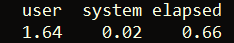Above, we’re able to calculate the same similarity scores in…under one second! This is vastly better than the first approach and is made possible due to the parallel operations vectorization performs under the hood. To see the randomly generated word with the maximum similarity score to “programming”, we can just run the below line of code:

```names(which.max(results))

```

This returns the string “wrrgrrmmrnb”.

That’s it for this post! Click here to view other R posts of mine.

The post Speed Test: Sapply vs. Vectorization appeared first on Open Source Automation.